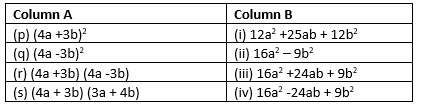# Important questions for Algebraic Expressions and Identities Class 8 Maths Chapter 9

In this page we have Important questions for Algebraic Expressions and Identities Class 8 Maths Chapter 9 .This page contains question on all format like subjective type questions, Multiple choice questions and Match the column. Hope you like them and do not forget to like , social share and comment at the end of the page.

## Subjective Type Questions

Question 1
Identify the terms, their coefficients for each of the following expressions.
(i)xyz2 + 3xy
(ii)1 - x -2x2
(iii)4p2q2 - 4p2q2r2+ r2
(iv)4 - xy + yz - xz
(v) (x/4) - (y/5) -y
(vi)1.3a - 2.6ab + 1.5b

(i) Terms xyz2, 3xy coefficients 1,3
(ii)Terms 1,-x,-2x2 Coefficients 1, -1,-2
(iii)Terms 4p2q2,-4p2q2r2,r2 Coefficients 4,-4,1
(iv) Terms 4,-xy,yz,-xz Coefficients 4,-1,1,-1
(v) Terms x/4, -y/5 ,-y Coefficients 1/4,-1/5,-1
(vi) Terms 1.3a,-2.6ab,1.5b Coefficients 1.3,-2.6 ,1.5

Question 2
Classify the following polynomials as monomials, binomials, trinomials. Which polynomials do not fit in any of these three categories?
(a)x2 + y2
(b)1000-x
(c)x + x2+ x3 + x4 + x5
(d)8 - y -5x
(e) 2y -3y2
(f) 2y - 3y+ 4y3
(g) 5x -8y + 3xy
(h) 4 -15z2
(i) ab + bc + cd + da+ 2ab
(j) pqr+2pq+5pqr
(k) p2q + pq2
(l) 2p + 2q+1

(a)x2 + y2: Binomial
(b)1000-x:Binomial
(c)x + x2+ x3 + x4 + x5:polynomial
(d)8 - y -5x:Trinomial
(e) 2y -3y2:Binomial
(f) 2y - 3y+ 4y3:Trinomial
(g) 5x -8y + 3xy:Trinomial
(h) 4 -15z2:Binomial
(i) ab + bc + cd + da+ 2ab:polynomial
(j) pqr+2pq+5pqr:Binomial
(k) p2q + pq2:Binomial
(l) 2p + 2q+1:Trinomial

Question 3
(i) ab - bc+ ac, bc - ca+ ab, ca - ab-2bc
(ii)p - q + pq, q - r + qr, r - p + pr , p+q+r
(iii)2p2q2- 3pq + 4, 5 + 7pq - 3p2q2 ,4p2q2 + 10pq
(iv)a2+ b2, b2+ c2, c2+ a2, 2ab + 2bc + 2ac

(i) ab - bc+ ac + bc - ca+ ab +ca - ab-2bc=ab-2bc+ac
(ii)p - q + pq + q - r + qr + r - p + pr + p+q+r=p+q+pq+r+qr+pr
(iii)2p2q2- 3pq + 4 + 5 + 7pq - 3p2q2 + 4p2q2 + 10pq=3p2q2+14pq+9
(iv) a2+ b2+ b2+ c2+ c2+ a2+ 2ab + 2bc + 2ac=2(a2+ b2+c2+ab+bc+ac)

Question 4.
(a) Subtract 8a - 7ab + 3b - 20 from 20a - 9ab + 5b - 20
(b) Subtract 3pq + 5qr - 7pr+1 from -4pq +2qr - 2pr + 5pqr+1
(c) Subtract 4p2q - 4pq - 5pq2 - 8p + 7q - 18
from 18 - 3p - 11q + 5pq - 2pq2+ 5p2q

While subtracting, we need to remember signs are reversed after �sign once bracket is opened ie. + becomes - and - becomes + Let solve the below question keeping that in mind (a) 20a - 9ab + 5b - 20 -(8a - 7ab + 3b - 20)=20a - 9ab + 5b - 20-8a +7ab-3b+20 =12a+2b -2ab
(b) -4pq +2qr - 2pr + 5pqr+1 -( 3pq + 5qr - 7pr+1 )=-4pq +2qr - 2pr + 5pqr+1 -3pq-5qr+7pr-1=-7pq -3qr+5pr+5pqr
(c) 18 - 3p - 11q + 5pq - 2pq2+ 5p2q -(4p2q - 4pq - 5pq2 - 8p + 7q - 18)= 18 - 3p - 11q + 5pq - 2pq2+ 5p2q -4p2q+4pq +5pq2+8p-7q+18=36-3p-18q+9pq+3pq2+5p2q -4p2q

Question 5
What are the coefficient of each term in the below expression?
4p2q2 + 4p2q2r2 - r2 +5
(a)4,4,-1,5
(b)4,4,1,5
(c) 4,4r2 ,-r2, 5
(d) None of these

## Multiple Choice Questions

Question 6
The product of a monomial and trinomial will be a
(a)monomial
(b)trinomial
(c)binomial
(d) None of these

Question 7
The exponents of a variable term in the polynomial is a
(a) integers
(b)negative integers
(c) positive integers
(d) non -negative integers

Question 8
The expression pqr +rqp+qpr is a
(a) Monomial
(b)trinomial
(c) binomial
(d) none of these

Answer is (a) as it sums to 3pqr

Question 9
Sum of a - b + ab, b + c - bc and c - a - ac is
(a) 2c + ab - ac - bc
(b) 2c - ab - ac - bc
(c) 2c + ab + ac + bc
(d) 2c - ab + ac + bc

Answer is (a) as it sums to 2c + ab � ac � bc

Question 10
The value of $(a + b)^2 + (a - b)^2$ is
(a) 2a + 2b
(b) 2a - 2b
(c) $2a^2 + 2b^2$
(d) $2a^2 - 2b^2$

Answer is (c) as $(a + b)^2 + (a - b)^2=a^2 + b^2 + 2ab +a^2 + b^2 - 2ab=2a^2 + 2b^2$

## Match the column

Question 11(p) -> (iii) (q) -> (iv) (r) -> (ii) (s) -> (i)

## Summary

This algebraic expressions and identities class 8 important questions is prepared keeping in mind the latest syllabus of CBSE . This has been designed in a way to improve the academic performance of the students. If you find mistakes , please do provide the feedback on the mail.